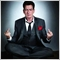• Übersicht
• Bewertungen (1)
• Diskussion

Martin new mt5

Grid Expert Advisor based on the martingale principle. Indicators included in the standard set of the terminal are used for performing deals. The EA features an interesting function of paired position closure for the first and last positions in the grid. A panel has been implemented on the chart to display certain values.

Description of Input Parameters

• Identification number - EA's ID number.
• Panel - enable/disable the panel on the chart
• Comment Deals - comment to deals
• Signal Strategy - select the strategy to perform deals
• Signal Start - enable/disable opening the first deal in a grid on a signal of the strategy
• Method calculation Volume - select the type of funds to calculate the volume of the first deal in a grid
• Risk - percentage of risk for the first deal in a grid; if set to zero, then the value from the Start Volume parameter is used
• Start Volume - volume of the first deal in a grid, if the Risk parameter is set to zero
• Type calculation Volume - method to calculate the volume of subsequent deals in the grid
• Coefficient calculation Volume - lot increase ratio, if the Type calculation Volume parameter is set to Multiplication or Addition
• Step calculation Volume - the number of deals, after which to increase the volume, if the Type calculation Volume parameter is set to Multiplication or Addition
• TakeProfit - take profit in pips, if the Dynamic Stop calculation parameter is disabled
• Distance - the minimum distance between orders, if the Dynamic Stop calculation parameter is disabled
• Slippage - slippage to send requests to the server to perform a deal
• Pairwise closing - enable/disable the paired closure of orders
• Dynamic Stop calculation - enable/disable the dynamic calculation of the TakeProfit and Distance parameters
• Period ATR - period of the ATR indicator to calculate the dynamic levels
• TimeFrames ATR - timeframe of the ATR to calculate the dynamic levels
• Coefficient TakeProfit - calculation coefficient of the TakeProfit parameter
• Coefficient Distance - calculation coefficient of the Distance parameter

Input parameters of the indicators for the corresponding strategies are intuitive.59
2018.02.25 17:33

8 instruments 2 days demo 1:500/ start 500 BGN, result 594 BGN + 18.8% worck good :)

Actual: robot work good, but 8 instsruments is very degerous. need trade max 2 instruments.